## 20141122

### Physics presentation: soundApparently the ultimate test of an impressive car sound system is not how loud it is, but is the subwoofer powerful enough to vibrate the air and shred a phonebook? (Video link: " MD style Paper Shredder !!!!.")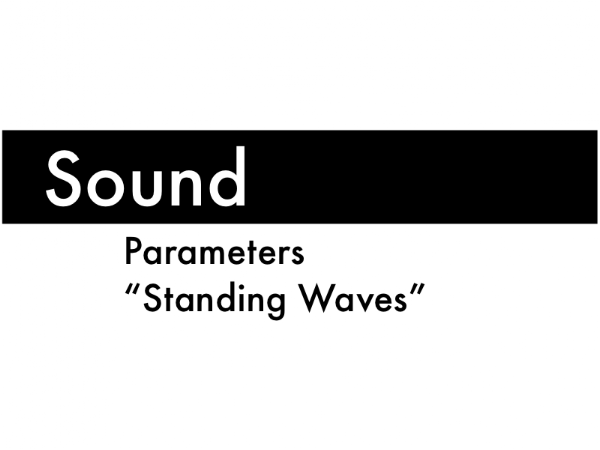In a previous presentation, we introduced wave parameters and standing waves along strings. Here we will extend these ideas to sound waves traveling in air.First, sound wave parameters.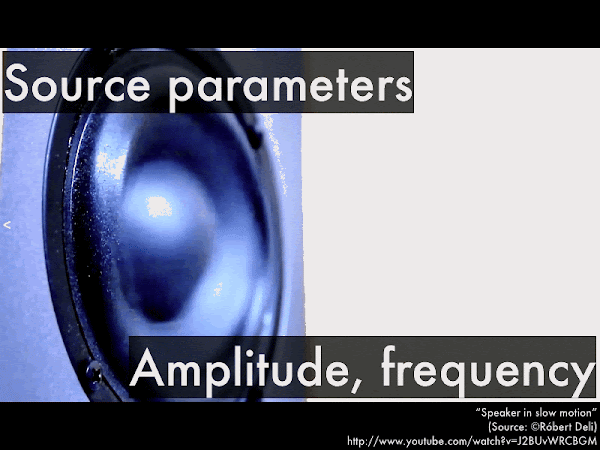The amplitude and the frequency of a sound wave is set by the source (here, a speaker cone) which will vibrate in and out, displacing the air in front of it.The amplitude of the speaker's in-and-out vibrations is related to the "loudness" of a sound--small amplitude vibrations barely displace the air molecules in front of the speaker from their nominal positions, while large amplitude vibrations will greatly displace the air molecules from their nominal positions. (The amount that the air is displaced will decrease as a sound wave spreads outwards from a source--thus making sounds "quieter" as you move further away from a source--but for the purposes of this presentation we'll ignore the spreading out of sounds.)The frequency of the speaker's in-and-out vibrations is related to the "pitch" of a sound--we perceive different frequency sounds in the range from 20 Hz (very low bass notes) up to 20,000 Hz (extremely high treble notes). These are nominal values, and typically the high end is lost due to age or unsafe sound exposure. (There can be sounds with frequencies lower than 20 Hz, if the source vibrates slowly enough--we humans can't hear these infrasound frequencies, but elephants can send out and receive these type of sounds over great distances to communicate with each other. Likewise if a source vibrates quickly enough, it will produce sounds with frequencies higher than 20,000 Hz--we humans can't hear these ultrasound frequencies, but bats can send out and receive these type of sounds, typically for navigating and preying on insects.)The next sound wave parameter is the speed that it propagates through air. Here we can see the spherical wavefront of the gun blast sound emitted from both the muzzle (and earlier wavefronts from the rear of the barrel). Note that the bullet is traveling faster than the speed of sound, resulting in a cone-shaped shock wave. More on that later.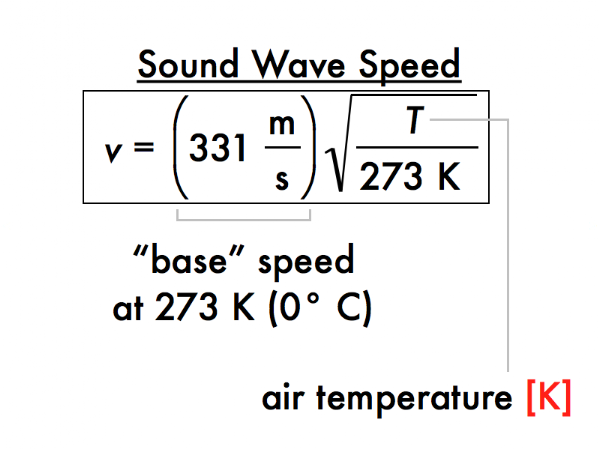The speed of a sound wave in air depends approximately on the square root of the absolute temperature (in kelvin, not in Celsius!), and at 273 K (0° C) would have a speed of 331 m/s, and at "room temperature" (according to some) of 293 K (20° C) sound waves would have a speed of:

v = (331 m/s)·sqrt((293 K)/(273 K)) = 342.91026079 m/s,

or to three significant figures, 343 m/s. (Some may define room temperature as high as 25° C, but apparently physicists like it a little cooler than some other people.) Essentially sound travels faster through warmer air, and slower through cooler air (neglecting changes in pressure). These sound waves are fast, but bullets and jets can certainly move faster than these speeds.Here, an F-14 Tomcat moving faster than the speed of sound creates that characteristic cone-shaped shock wave, which to an observer sounds as a sonic boom. (Video link: "F-14_Tomcat_sonic_boom.ogg.")The speed of a sound wave is set by the temperature of the medium (air), but the frequency of the wave is set by the source. The resulting spatial repeat interval is the wavelength, which is the distance between the "crowding" wavefronts. Note that while a single air molecule (highlighted in yellow) just moves back-and-forth (at the average random speed of an ideal gas at that temperature), the wavefronts of a wave travel from left-to-right at the speed of sound.(This is a review of our previous discussion of one-dimensional rope and string waves.) Note the hierarchy of these wave parameters. Since the wave speed is determined by properties of the material it travels through (independent of the source), and the frequency is determined by the source (independent of the medium), these are said to be independent wave parameters. In contrast, the wavelength of the wave is dependent on both the independent speed and frequency parameters. Algebraically there is nothing wrong with expressing this relation as v = λf and f = v/λ, as long as you recognize that the dependency of λ doesn't change.The speaker on the left oscillates back-and-forth as the source of a sound wave that travels left-to-right along this section of air. The top case is where the speaker oscillates back-and-forth with a certain frequency and a small amplitude, while the bottom case is where the speaker oscillates back-and-forth with the same frequency and larger amplitude. Which wave travels with the faster speed? Which wave has the longer wavelength?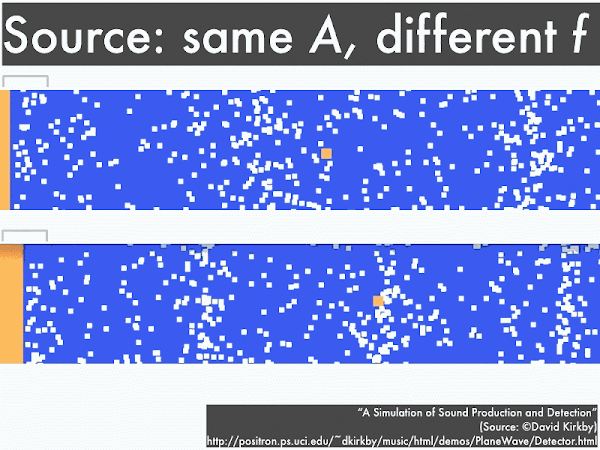Here both speakers oscillate back-and-forth with the same amplitude, but the top case has a lower frequency and the bottom case has a higher frequency. Which wave travels with the faster speed? Which wave has the longer wavelength?In this setup, the a speaker oscillates back-and-forth with a given frequency and amplitude, creating the waves in the first section at left. As the wave travels from left-to-right, it is then transferred to the second section that allows the wave to travel at a much slower speed (perhaps due to a cooler air temperature, or a different gas density). Along which section does the wave have a higher frequency? Along which section does the wave have a longer wavelength?Now we'll briefly extend our previous discussion on standing waves on strings to sound standing waves in pipes.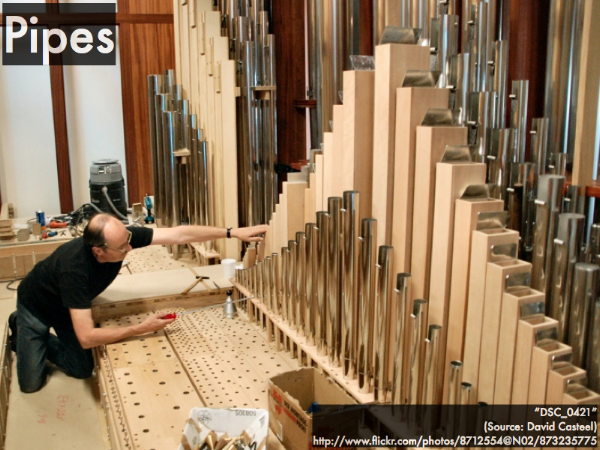Sound waves can be "trapped" inside pipes of various lengths, with either open and/or closed ends, and if a sound wave matches the resonant frequency of the pipe, then the air inside will move back-and-forth in a coordinated manner--a "standing wave."We can regard a pipe with both ends closed, or a pipe with both ends open as a "symmetric" system. If they have the same length, then the air molecules will resonate with the same fundamental frequency (in this animation, approximately 1 Hz). Note that while the standing waves have the same frequency for both pipes, the closed-closed pipe has an antinode in the middle, where air molecules slosh back-and-forth, while there are nodes at either end, where air molecules are "trapped" because they cannot move into (against) the closed ends.

In contrast, the open-open pipe has antinodes at either end, as air molecules are free to slosh back-and-forth, being exposed to the atmosphere, while there is a node in the middle, where air molecules are "trapped" because of the motion at either end.

Because the distance between consecutive nodes and antinodes in these pipes is proportional to the wavelength of the standing wave, the fundamental frequency would be the same for either closed-closed or open-open pipes.For a closed-closed or open-open symmetric pipe, the frequency at which a source of sound waves would resonate within this pipe are multiples of the fundamental frequency f1, which depends on the wave speed v of sound (343 m/s for "room temperature"), and the length of the pipe.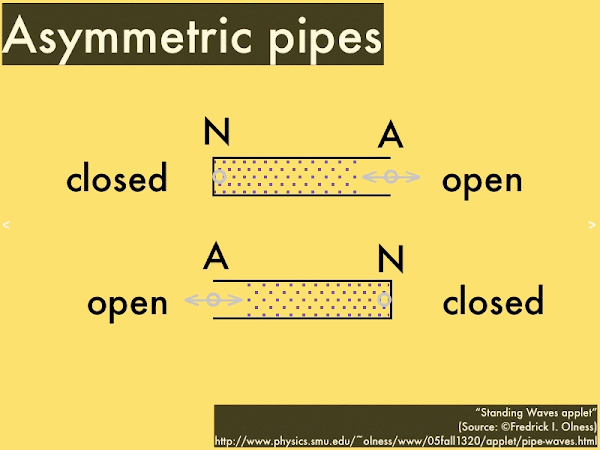We can regard a pipe with one end open, while the other end is closed (or vice versa) as a "symmetric" system. If they have the same length, then the air molecules will resonate with the same fundamental frequency (in this animation, approximately 2 Hz). Note that while the standing waves have the same frequency for both pipes, the open end has an antinode, where air molecules exposed to the atmosphere slosh back-and-forth, while the closed end has a node, where air molecules are "trapped" because they cannot move into (against) the closed ends.

(Because the distance between consecutive nodes and antinodes in these pipes is proportional to the wavelength of the standing wave, the fundamental frequency would be the same for either closed-open or open-closed pipes. Note for a given length of pipe, the asymmetric systems have a longer (bigger value) node-antinode spacing than the symmetric systems, and thus an asymmetric system would have a lower (smaller value) fundamental frequency (in this animation, approximately 2 Hz) than the symmetric system.)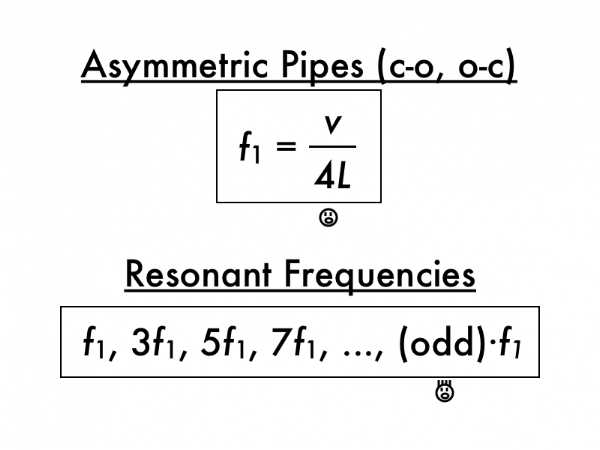For a closed-open or open-closed asymmetric pipes, the fundamental frequency is lower than a comparable symmetric pipe (note the factor of 4 instead of 2 in the denominator), and resonant frequencies are all odd multiples of the fundamental frequency (rather than integer multiples). How very, very...odd. But this follows from the asymmetry of closed-open and open-closed pipes, such that even standing wave frequency multiples are not allowed.Let's close out with some trombone playing, which can be approximated as a symmetric pipe (the bell end is open to the air, while the mouthpiece end is open to your lungs). In order to play different notes on a trombone is to buzzing your lips, not just at any arbitrary frequency, but only at multiples of the fundamental frequency in order to resonate the air along the pipe. Higher resonant frequency notes can be accomplished either by changing the length L of the trombone by shortening the slide... (Video link: "How to play the Trombone B Flat Major Scale.")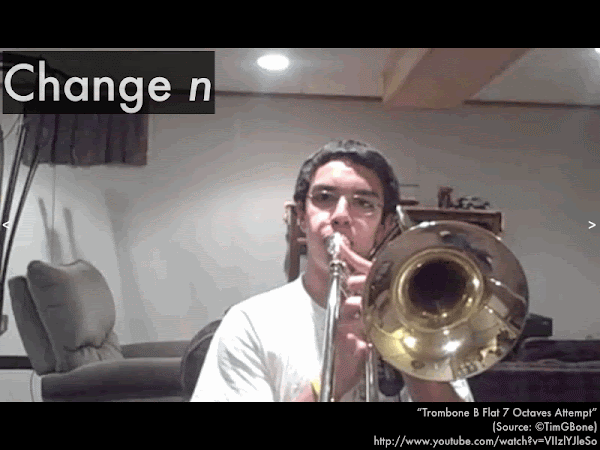...or by keeping the position of the slide fixed (such that L is constant), and buzzing your lips to match higher resonant frequency notes. (Video link: "Trombone B Flat 7 Octaves Attempt.") This takes a fair amount of manual and lip coordination, so keep that in mind the next time you are subjected to a novice trombone player practicing the scales.